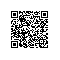# 利用递归查找目录中的最小和最大文件（包括子目录）

package file;

import java.io.File;

public class TestFile5 {

private static File minFile, maxFile;

public static void main(String[] args) {
File root = new File("d:/test");
findMinAndMax(root);
}

public static void findMinAndMax(File rootDirectory) {
File[] fs = rootDirectory.listFiles();
for (File f : fs) {
if (f.isDirectory())
findMinAndMax(f);
else {
if (minFile == null) {
minFile = maxFile = f;
}
if (f.length() != 0) {
if (f.length() < minFile.length())
minFile = f;
if (f.length() > maxFile.length())
maxFile = f;
}
}
}
}
}使用钉钉扫一扫加入圈子
+ 订阅